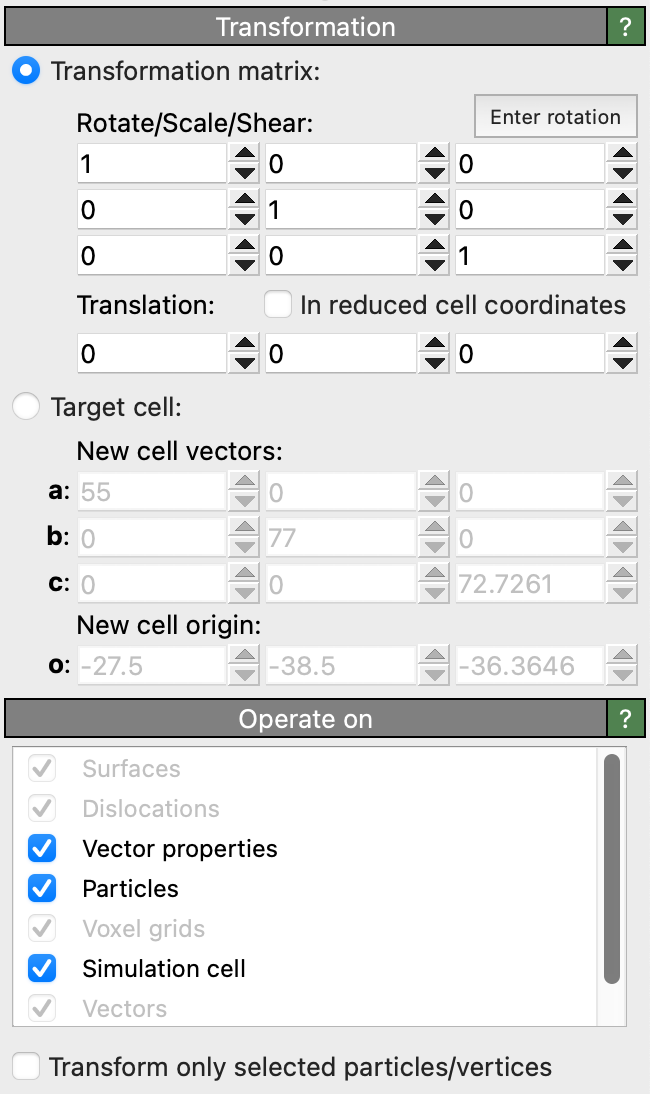# Affine transformationThis modifier applies an affine transformation to the system or specific parts of it. It may be used to translate, scale, rotate or shear the particles, the simulation cell and/or other elements. The transformation can either be specified explicitly in terms of a 3x3 matrix plus a translation vector, or implicitly by prescribing a fixed target shape for the simulation cell.

Given a 3x3 linear transformation matrix $$\mathbf{M}$$ and a translation vector $$\mathbf{t}$$, which together describe a general affine transformation, the transformed position of a particle at the original Cartesian position $$\mathbf{x}$$ is computed as $$\mathbf{x}' = \mathbf{M} \cdot \mathbf{x} + \mathbf{t}$$. This notation uses column vectors.

The button Enter rotation opens a dialog box which lets you specify a rotation axis, a rotation angle and a center of rotation. Based on these inputs, OVITO computes the corresponding affine transformation for you.

## Translation in reduced coordinates

The option In reduced cell coordinates changes the affine transformation method such that the translation vector $$\mathbf{t}$$ is specified in reduced cell coordinates instead of Cartesian coordinates, i.e. in terms of the three vectors that span the simulation cell (after they have been transformed by the linear matrix $$\mathbf{M}$$).

In other words, activating this option changes the affine transformation equation to $$\mathbf{x}' = \mathbf{M} \cdot (\mathbf{x} + \mathbf{H} \cdot \mathbf{t})$$ with $$\mathbf{H}$$ being the 3x3 cell matrix formed by the three edge vectors of the original simulation cell.

## Transform to target cell

The Transform to target cell option lets the modifier dynamically compute the affine transformation based the original shape of the simulation cell and a specified target cell shape. The automatically determined transformation maps the existing simulation cell exactly onto the given target cell shape. All contents of the simulation cell (e.g. particles, surface meshes, etc.) will be mapped into the new cell shape accordingly, unless you turn off their transformation (see next section).

Note that you can use this option to replace a varying simulation cell loaded from the input trajectory file(s) with a constant cell shape of your choice.

## Transformed elements

You can select the types of data elements that should get transformed by the modifier:

Element type

Description

Particles

Applies the affine transformation to the coordinates of particles (i.e. the Position particle property).

Vector particle properties

Applies the linear part $$\mathbf{M}$$ of the affine transformation to the Velocity, Force and Displacement particle properties.

Simulation cell

Applies the affine transformation to the origin of the simulation cell and the linear part to the three cell vectors.

Surfaces

Applies the affine transformation to the vertices of surface meshes and triangle meshes.

Voxel grids

Applies the affine transformation to the domain shape of a voxel grid.

Dislocations

Applies the affine transformation to a set of dislocation lines.

The option Transform selected elements only restricts the application of the transformation to the currently selected particles.